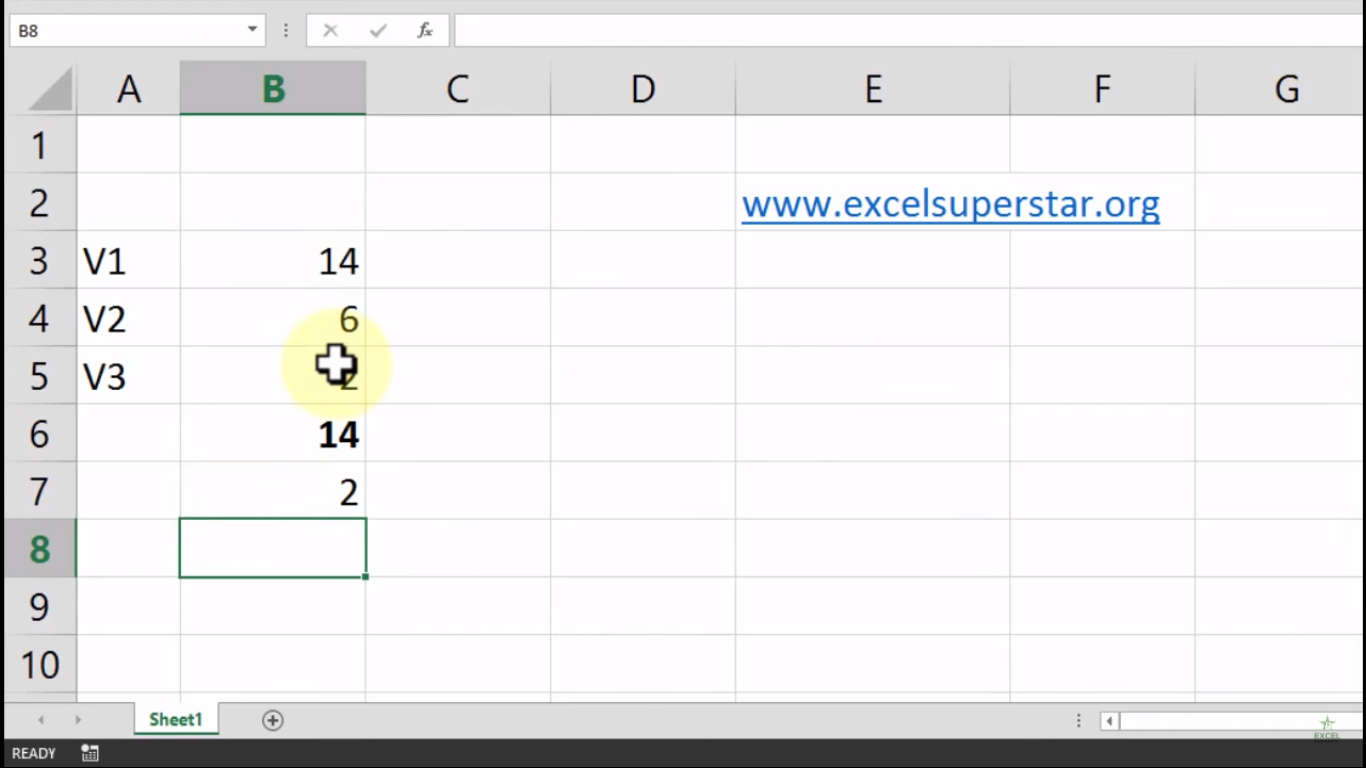# Excel MAX FunctionIn this tutorial we will learn about the use of MAX function in Excel.

Example: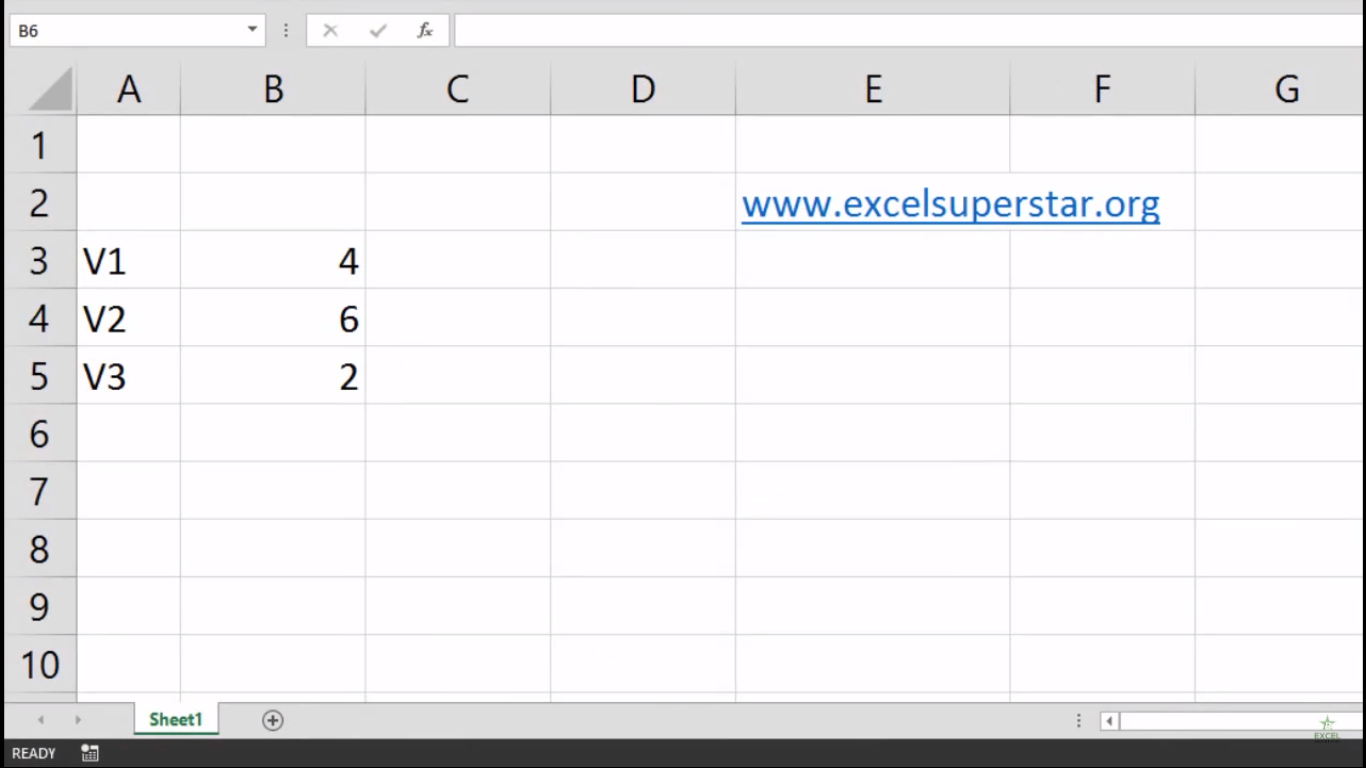I have a report card in which there are 2 columns consist of Subjects and total marks allotted in each subject. Now from the total marks column we have to calculate the maximum value scored in a subject

So to calculate the maximum value we will be applying Max Function in Excel.

1. Write =max and press the Tab Key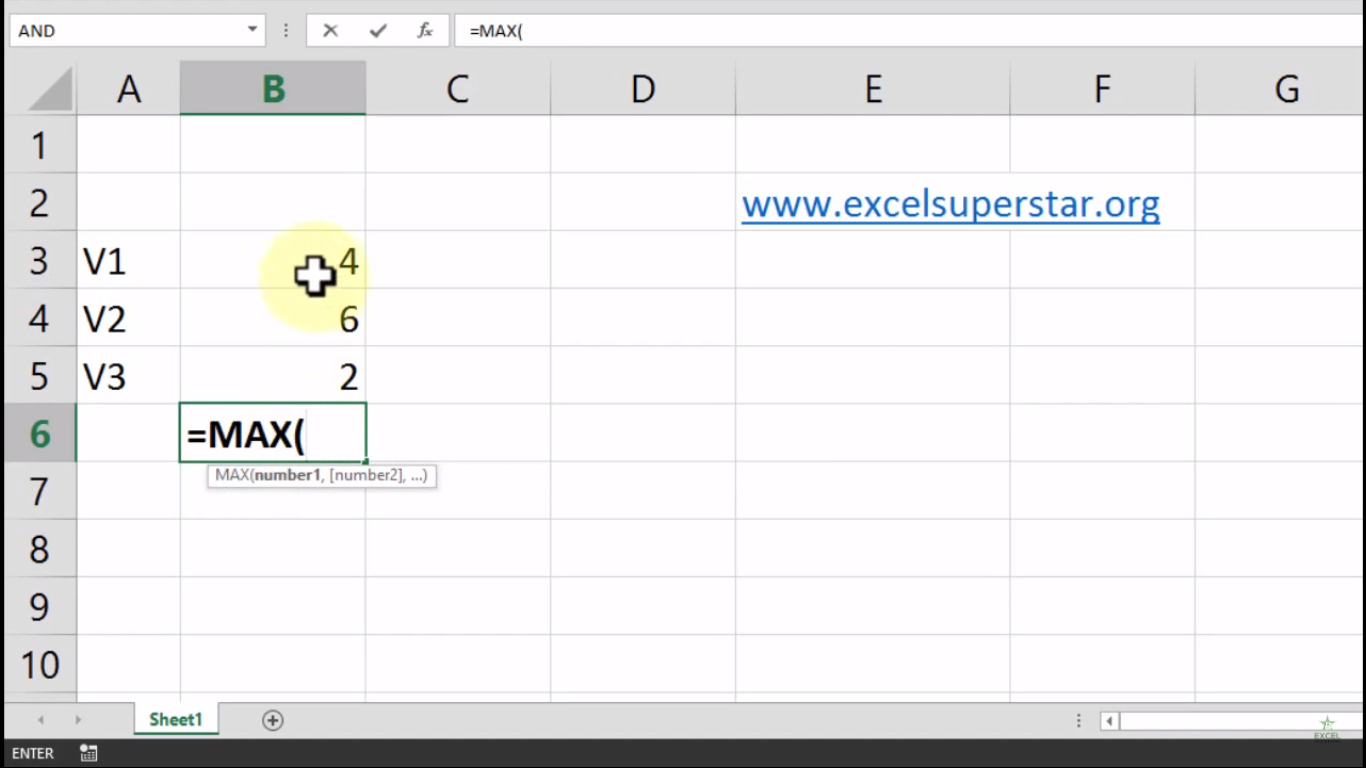2. Select the entire range =MAX(B3:B5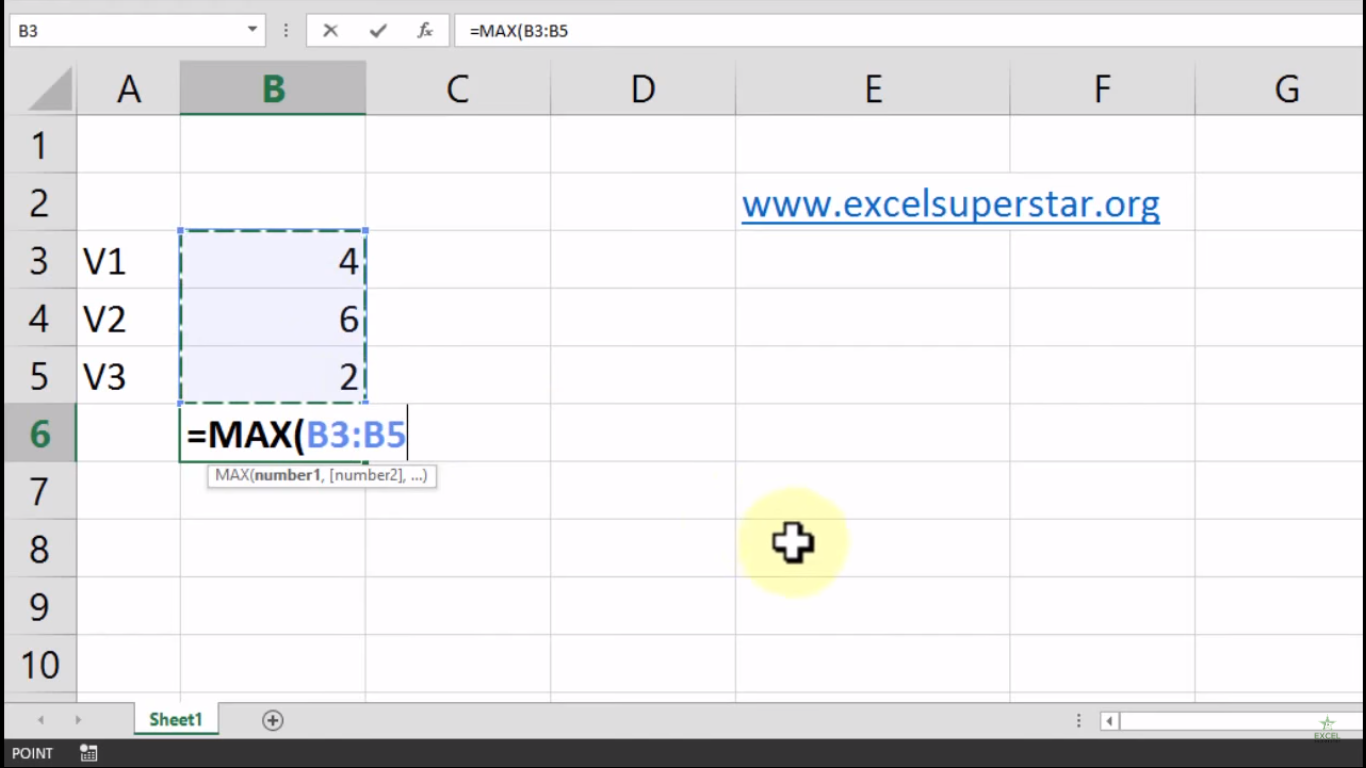3. Close the bracket and press Enter =MAX(B3:B5)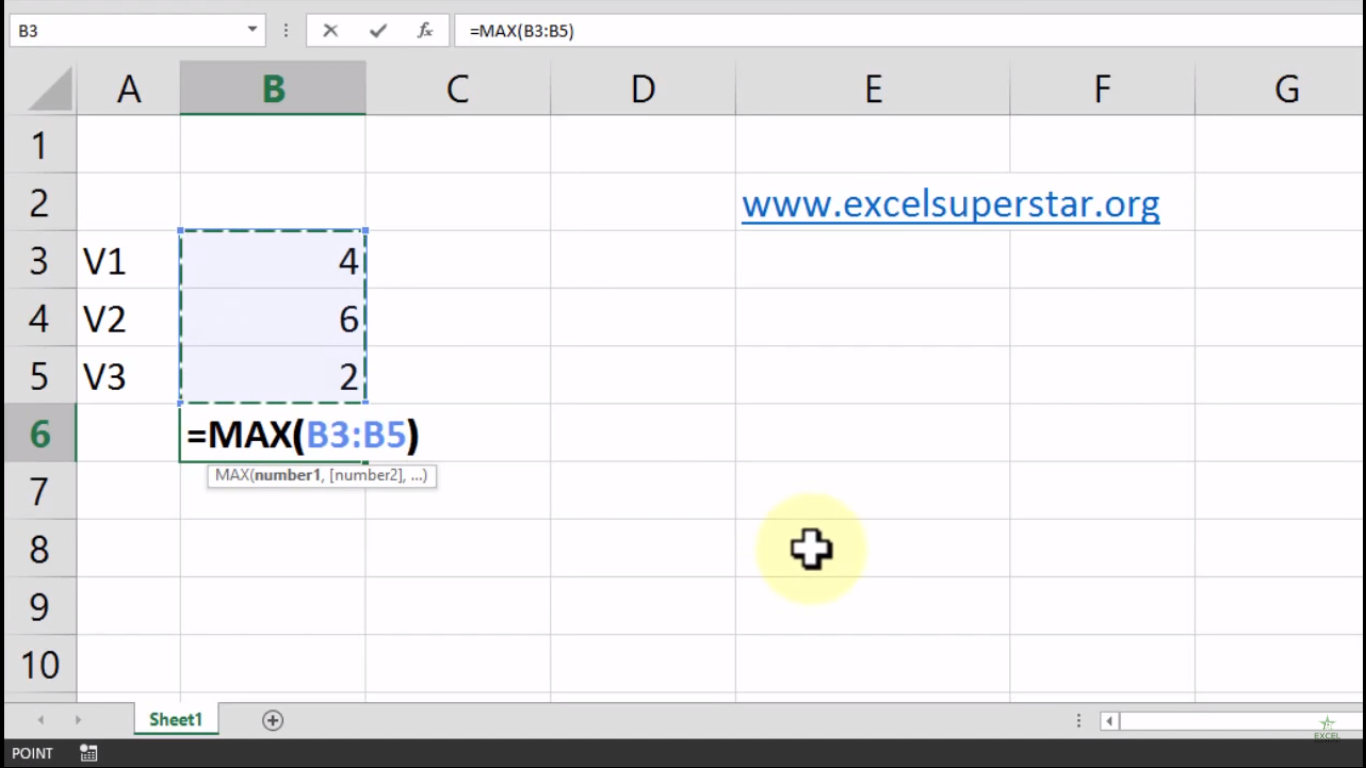4. The maximum value is 6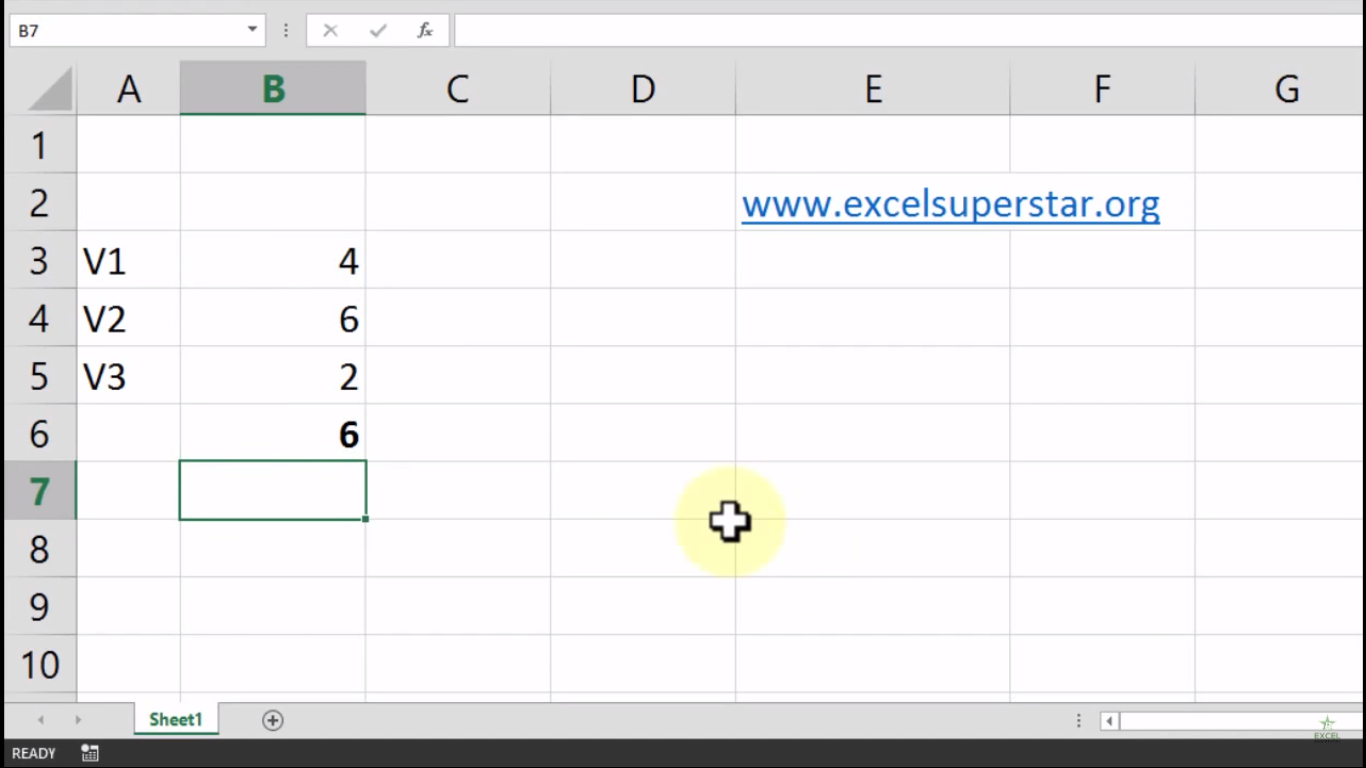If you are looking to find minimum value from the columns then we have to apply MIN Formula in Excel by following the steps given below:

1. Write =min and press the Tab Key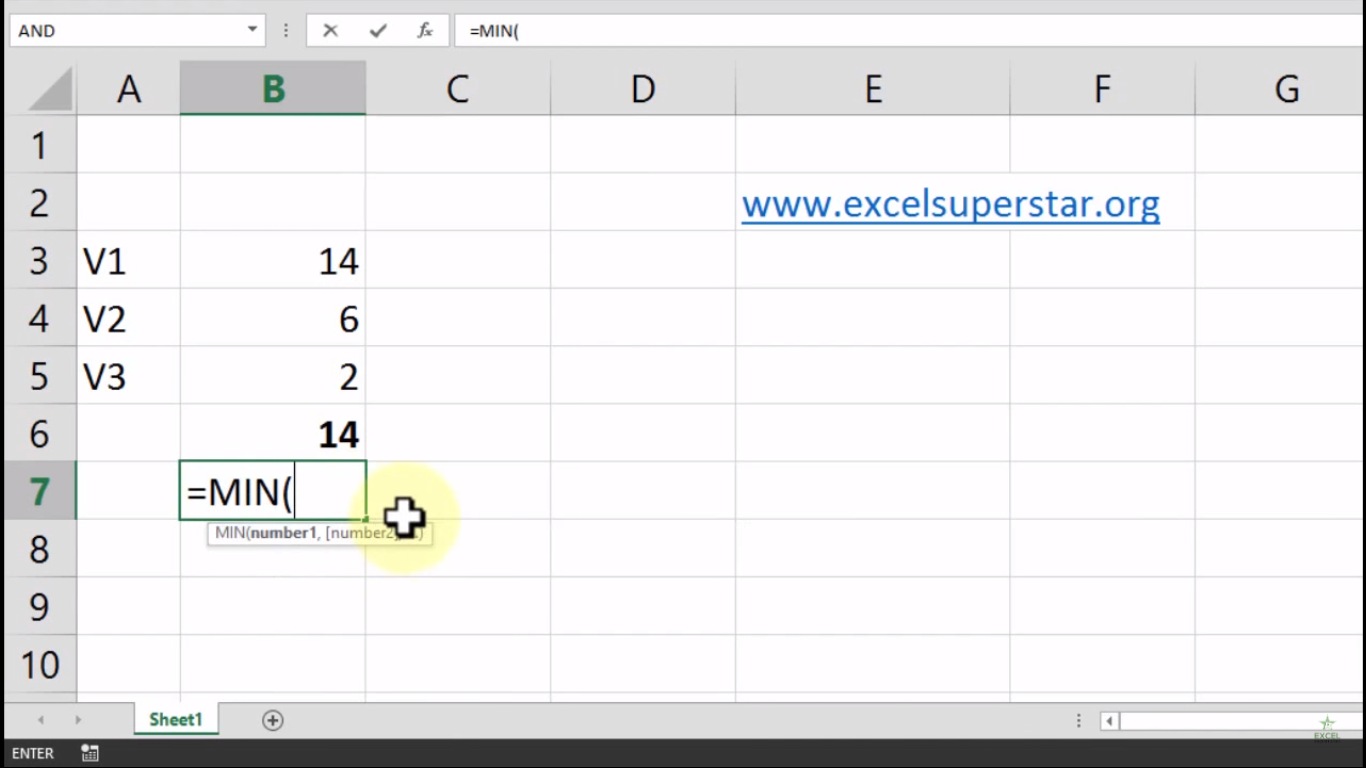2. Select the entire range =MIN(B3:B5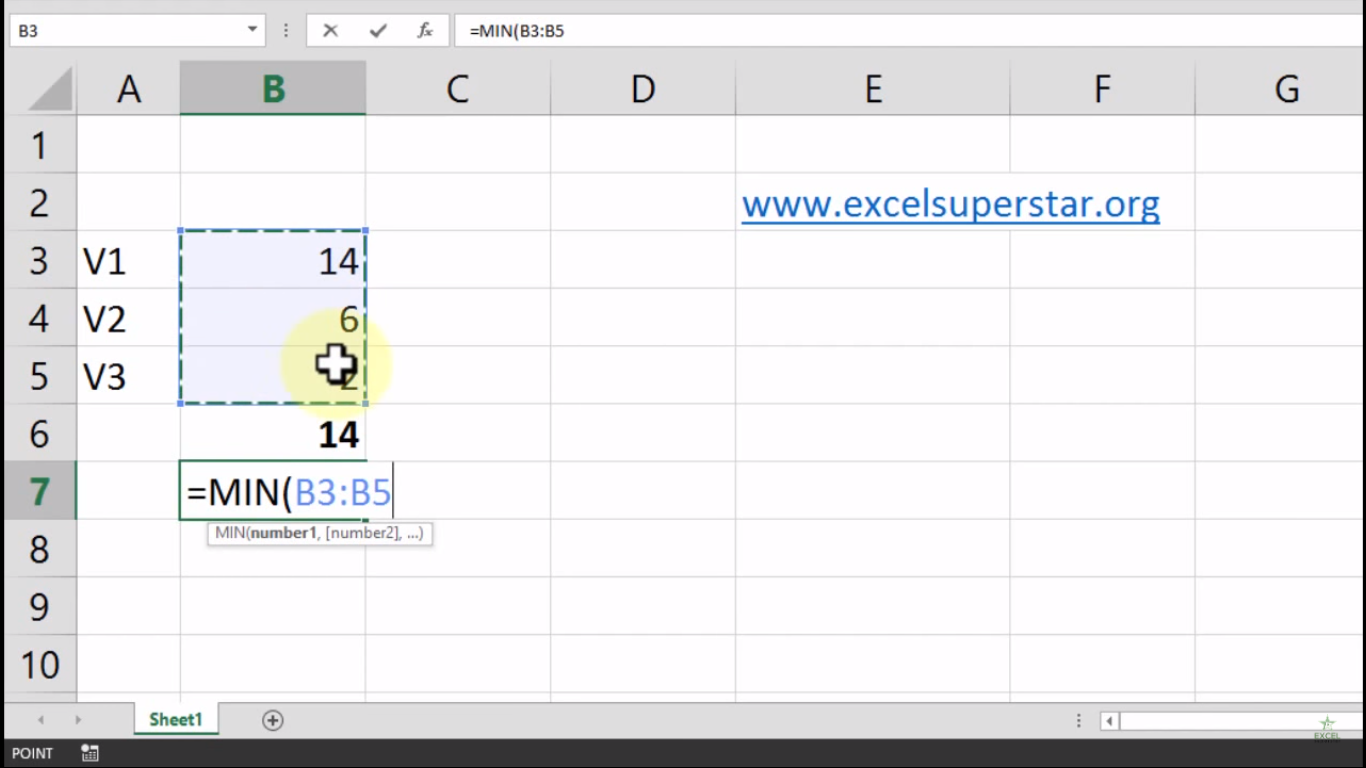3. Close the bracket and press Enter =MIN(B3:B5)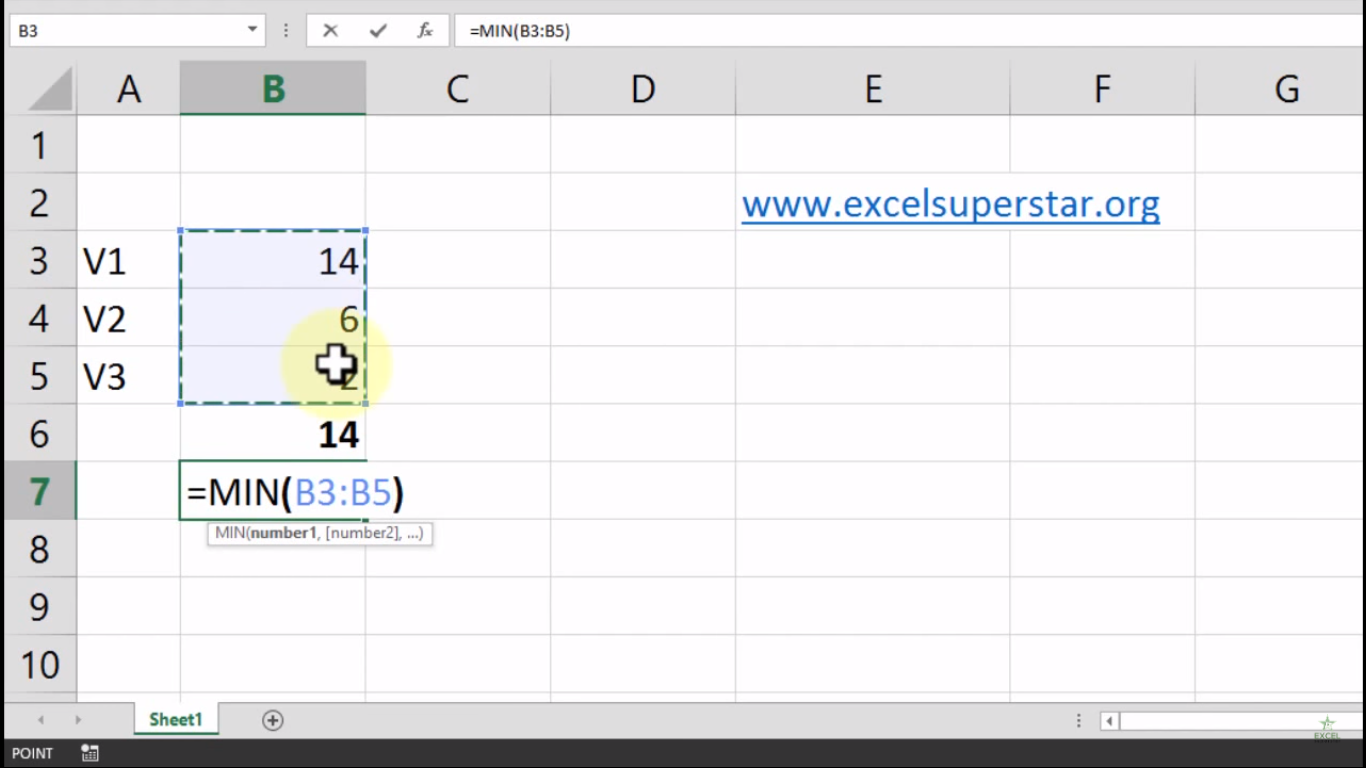4. The minimum value is 2